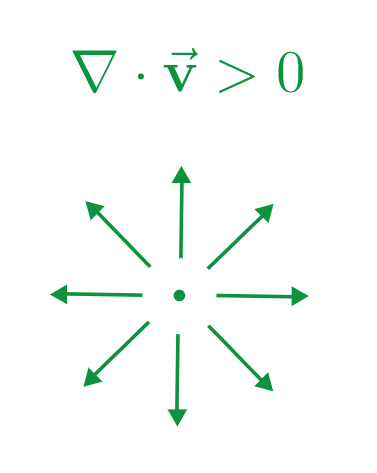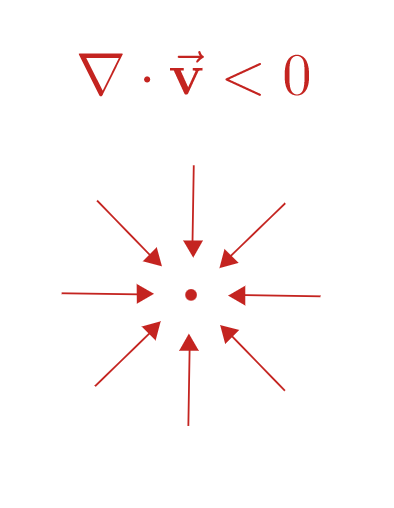Uh Oh! It seems you’re using an Ad blocker!

Since we’ve struggled a lot to makes online calculations for you, we are appealing to you to grant us by disabling the Ad blocker for this domain.# Divergence Calculator

$$\mathbf{\vec{F}}\left(x,y,z\right)$$:

x:

y:

z:

$$\left(x_{0}, y_{0}, z_{0}\right)$$: (optional)

$$x_{0}$$:

$$y_{0}$$:

$$z_{0}$$:

Table of Content

 1 What is Divergence? 2 Types of Divergence: 3 Divergence Formula: 4 How to Calculate Divergence? 5 What is a real-life example of the divergence phenomenon? 6 What does the divergence theorem tell us? 7 Is divergence the same as flux? 8 What is meant by curl?

Get the Widget!

Add this calculator to your site and lets users to perform easy calculations.

Feedback

How easy was it to use our calculator? Did you face any problem, tell us!

An online divergence calculator is specifically designed to find the divergence of the vector field in terms of the magnitude of the flux only and having no direction. Just like a curl of a vector field, the divergence has its own specific properties that make it a valuable term in the field of physical science.

If you are interested to know more about the physical phenomenon of this term, you are on the right platform.

## What is Divergence?

In the dictionary of vector analysis:

“A vector operator that actually measures the norm of the source and sink of the field in terms of a signed scalar is called divergence”

### Types of Divergence:

Depending upon the flow of the flux, the divergence of a vector field is categorized into two types:

Positive Divergence:

The point from which the flux is going in the outward direction is called positive divergence. The point is known as the source.Negative Divergence:

The point from which the flux is going in the inward direction is known as negative divergence. Here, the point acts as a sink.Zero Divergence:

Zero divergence means that nothing is being lost. In other words, the amount of the flux coming is equivalent to that of the flux going.You can identify each and every type of divergence instantly by using our free online divergence calculator.

### Divergence Formula:

Calculating divergence of a vector field does not give a proper direction of the outgoingness. However, the following mathematical equation can be used to illustrate the divergence as follows:

Divergence= ∇ . A

As the operator delta is defined as:

$$∇ = \frac{\partial}{\partial x}P, \frac{\partial}{\partial y}Q, \frac{\partial}{\partial z}R$$

So the formula for the divergence is given as follows:

$$Divergence of {\vec{A}} = \left(\frac{\partial}{\partial x}P, \frac{\partial}{\partial y}Q, \frac{\partial}{\partial z}R\right)\cdot {\vec{A}}$$

### How to Calculate Divergence?

You can use our free online divergence calculator to obtain more accurate results, but it is very crucial to get hands-on practice on a few examples to understand the basic concept of divergence of a vector field. Let’s move on!

Example # 01:

Find the divergence of the vector field represented by the following equation:

$$A = \cos{\left(x^{2} \right)},\sin{\left(x y \right)},3$$

Solution:

As we know that the divergence is given as:

Divergence= ∇ . A

or;

$$Divergence of {\vec{A}} = \left(\frac{\partial}{\partial x}, \frac{\partial}{\partial y}, \frac{\partial}{\partial z}\right)\cdot {\vec{A}}$$

By putting the values, we get:

$$Div {\vec{A}} = \left(\frac{\partial}{\partial x}, \frac{\partial}{\partial y}, \frac{\partial}{\partial z}\right)\cdot \left(\cos{\left(x^{2} \right)},\sin{\left(x y \right)},3\right)$$

$$Div {\vec{A}}= \frac{\partial}{\partial x} \left(\cos{\left(x^{2} \right)}\right) + \frac{\partial}{\partial y} \left(\sin{\left(x y \right)}\right) + \frac{\partial}{\partial z} \left(3\right)$$

$$Div {\vec{A}} = \frac{\partial}{\partial x} \left(\cos{\left(x^{2} \right)}\right) + \frac{\partial}{\partial y} \left(\sin{\left(x y \right)}\right) + \frac{\partial}{\partial z} \left(3\right)$$

Now, we need to determine the partial derivatives of each term separately:

$$\frac{\partial}{\partial x} \left(\cos{\left(x^{2} \right)}\right) = – 2 x \sin{\left(x^{2} \right)}$$

$$\frac{\partial}{\partial y} \left(\sin{\left(x y \right)}\right) = x \cos{\left(x y \right)}$$

$$\frac{\partial}{\partial z} \left(3\right) = 0$$

(click partial derivative to get step by step calculations)

Calculating divergence as a sum of all the terms:
$$Div {\vec{A}} = \left(- 2 x \sin{\left(x^{2} \right)}+x \cos{\left(x y \right)}+0\right)$$

You may also like to use our free divergence of vector field calculator to determine the flow of a fluid or a gas in terms of magnitude.

Example # 02:

Calculate divergence of the vector field given below:

$$B = \sin{\left(x \right)},\cos{\left(y \right)},2 z$$

Solution:

The divergence of a vector field is illustrated as:

Divergence= ∇ . A

or;

$$Divergence of {\vec{A}} = \left(\frac{\partial}{\partial x}, \frac{\partial}{\partial y}, \frac{\partial}{\partial z}\right)\cdot {\vec{A}}$$

So, we have:

$$Divergence of {\vec{A}} = \left(\frac{\partial}{\partial x}, \frac{\partial}{\partial y}, \frac{\partial}{\partial z}\right)\cdot \left(\sin{\left(x \right)}, \cos{\left(y \right)}, 2 z\right)$$

Writing each term separately with its partial derivative:

$$Divergence of {\vec{A}} = \frac{\partial}{\partial x} \left(\sin{\left(x \right)}\right) + \frac{\partial}{\partial y} \left(\cos{\left(y \right)}\right) + \frac{\partial}{\partial z} \left(2 z\right)$$

Taking partial derivatives of each term individually:

$$\frac{\partial}{\partial x} \left(\sin{\left(x \right)}\right) = \cos{\left(x \right)}$$

$$\frac{\partial}{\partial y} \left(\cos{\left(y \right)}\right) = – \sin{\left(y \right)}$$

$$\frac{\partial}{\partial z} \left(2 z\right) = 2$$

(click partial derivative to get step by step calculations)

Now, calculating divergence by summing up all the terms as follows:

$$Divergence of {\vec{A}} = \cos{\left(x \right)}+ \sin{\left(y \right)}+2$$

However, if you use our free online divergence calculator, the chances of any uncertainty are reduced.

### How Divergence Calculator Works?

Our calculator is best among all the calculators that are used to find the divergence of the vector field.

Let us see what to do!

Input:

• Write the values against each coordinate of the vector field that is given

Now, if you want to find divergence for a certain coordinate:

• Write down the desired coordinate value
• Click ‘calculate’

Output:

The free divergent calculator calculates:

• Partial derivatives of each term involved in the formula
• Sum up all the values to give divergence of the field given
• Step by step calculations to better get the idea

## FAQ’s:

### What is a real-life example of the divergence phenomenon?

In a real atmosphere, divergence occurs when a strong iwing=d moves away from the weaker wind. When divergence occurs in the upper levels of the atmosphere, it leads to rising air.

### What does the divergence theorem tell us?

This theorem states that if you use a triple integral for a divergence to determine the sum of little bits outward flow in a volume, you will get a total outward flow for that volume. To determine this flow, you can use our divergence theorem calculator for free.

### Is divergence the same as flux?

Yes, you can subject the divergence of a vector field as its flux density entering or leaving a point that can be measured easily with the help of a free online divergence of a vector calculator.

### What is meant by curl?

The angular rotation of the flux about a point in a specific direction is called curl of a vector field.

## Conclusion:

Divergence tells us how the strength of a vector field is changing instantaneously. We can see a vast use of the divergence theorem in the field of partial differential equations where they are used to derive the flow of heat and conservation of mass. However, our free online divergence calculator provides you with the ease to determine the divergence of a vector field more accurately.

## Reference:

From the source of Wikipedia: Informal derivation, Gauss’s law, Ostrogradsky instability.

From the source of khan academy: Intuition for divergence formula, rotation with a vector.

From the source of lumen learning: Vector Fields, Path Independence, Line Integrals, Green’s Theorem, Curl and Divergence.